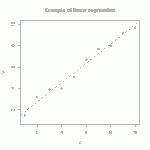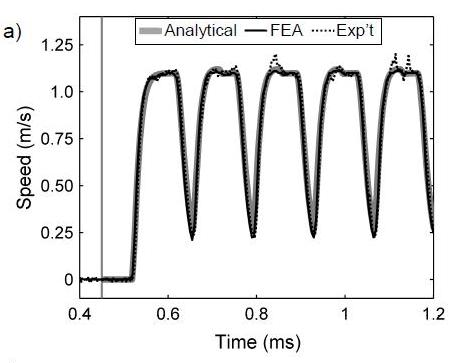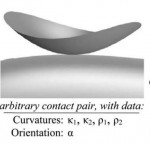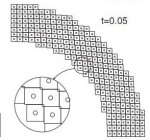# Truss myths and analysis

I’ve shared my undergrad lecture slides on “myths in truss analysis” so often that I’m making my life easier by now sharing them more broadly: http://csm.mech.utah.edu/TrussMythsAndTrussExamples.pptx. Please let me know if you see errors!

# Annulus Twist as a verification test

Illustrated below is the solution to an idealized problem of a linear elastic annulus (blue) subjected to twisting motion caused by rotating the T-bar an angle$\alpha$.  The motion is presumed to be applied slowly enough that equilibrium is satisfied.This simple problem is taken to be governed by the equations of equilibrium$\vec{\nabla}\cdot\sigma=0$, along with the plane strain version of Hooke’s law in which Cauchy stress is taken to be linear with respect to the small strain tensor (symmetric part of the displacement gradient).  If this system of governing equations is implemented in a code, the code will give you an answer, but it is up to you to decide if that answer is a reasonable approximation to reality. This observation helps to illustrate the distinction between verification (i.e., evidence that the equations are solved correctly) and validation (evidence that physically applicable and physically appropriate equations are being solved).  The governing equations always have a correct answer (verification), but that answer might not be very predictive of reality (validation).

# Resource: Perfect triples, “nice” unit vectors, and “nice” orthogonal matrices

“NICE” lists:

Have you ever noticed that textbooks often involve so-called 3-4-5 triangles? They do that to make the algebraic manipulations easier for students.  If the two legs of a right triangle are of length 3 and 4, then the hypotenuse (found from the Pythagorean theorem) has a length of 5, which is “nice” in the sense that it is an integer rather than an irrational square root that more typically comes from solving the Pythagorean theorem. As discussed in many elementary math sites (such as MakingMathematics.org), another example of a “nice” triangle is the 5-12-13 triangle, since$5^2+12^2=13^2$.

The external links in this posting contain a list of more of these so-called perfect triples of integers$\{a,b,c\}$ for which$a^2+b^2=c^2$. Perfect triples are also used to create “nice” 2D unit vectors whose components are each rational numbers (instead of involving irrational square roots from the normalization process). For example, the classic unit vector based on the 3-4-5 perfect triple is simply$\{\frac{3}{5},\frac{4}{5}\}$. Continue reading

# Tutorial: multi-linear regressionThe straight line is the linear regression of a function that takes scalars (x-values) as input and returns scalars (y-values) as output. (figure from GANFYD)

You’ve probably seen classical equations for linear regression, which is a procedure that finds the straight line that best fits a set of discrete points$\{(x_1,y_1), (x_2,y_2),...,(x_N,y_N)\}$. You might also be aware that similar formulas exist to find a straight line that is a best (least squares) fit to a continuous function$y(x)$.The pink parallelogram is the multi-linear regression of a function that takes vectors (gray dots) as input and returns vectors (blue dots) as output

The bottom of this post provides a link to a tutorial on how to generalize the concept of linear regression to fit a function$\vec{y}(\vec{x})$ that takes a vector$\vec{x}$ as input and produces a vector$\vec{y}$ as output. In mechanics, the most common example of this type of function is a mapping function that describes material deformation: the input vector is the initial location of a point on a body, and the output vector is the deformed location of the same point. The image shows a collection of input vectors (initial positions, as grey dots) and a collection of output vectors (deformed locations as blue dots). The affine fit to these descrete data is the pink parallelogram. Continue reading

# Course offering: ME 7960 (special topics) Computational Constitutive ModelingConstitutive modeling refers to the development of equations describing the way that materials respond to various stimuli. In classical deformable body mechanics, a simple constitutive model might predict the stress required to induce a given strain; the canonical example is Hooke’s law of isotropic linear elasticity. More broadly, a constitutive model predicts increments in some macroscale state variables of interest (such as stress, entropy, polarization, etc.) that arise from changes in other macroscale state variables (strain, temperature, electric field, etc.).

Constitutive equations are ultimately implemented into a finite element code to close the set of equations required to solve problems of practical interest. This course describes a few common constitutive equations, explaining what features you would see in experimental data or structural behavior that would prompt you to select one constitutive model over another, how to use them in a code, how to test your understanding of the model, how to check if the code is applying the model as advertised in its user’s manual, and how to quantitatively assess the mathematical and physical believability of the solution.

# Presentation: Contact Mechanics of Impacting Slender Rods: Measurement and Analysis

Sanders, A., I. Tibbitts, D. Kakarla, S. Siskey, J. Ochoa, K. Ong, and R. Brannon. (2011). “Contact mechanics of impacting slender rods: measurement and analysis.” Society for Experimental Mechanics Annual Meeting. Uncasville, CT, June 13-16.

Abstract

To validate models of contact mechanics in low speed structural impact, slender rods with curved tips were impacted in a drop tower, and measurements of the contact and vibration were compared to analytical and finite element (FE) models. The contact area was recorded using a thin-film transfer technique, and the contact duration was measured using electrical continuity. Strain gages recorded the vibratory strain in one rod, and a laser Doppler vibrometer measured velocity. The experiment was modeled analytically using a quasi-static Hertzian contact law and a system of delay differential equations. The FE model used axisymmetric elements, a penalty contact  algorithm, and explicit time integration. A small submodel taken from the initial global model economically refined the analysis in the small contact region. Measured contact areas were within 6% of both models’ predictions, peak speeds within 2%, cyclic strains within 12 microstrain (RMS value), and contact durations within 2 µs. The accuracy of the predictions for this simple test, as well as the versatility of the diagnostic tools, validates the theoretical and computational models, corroborates instrument calibration, and establishes confidence thatthe same methods may be used in an experimental and computational study of the impact mechanics of artificial hip joint.

Available Online:Global model results comparison with analytical and experimental results for speed at the midpoint of one of the rods

# Publication: Determining a Surrogate Contact Pair in a Hertzian Contact Problem

Sanders, A. P. and R. M. Brannon (2011). “Determining a Surrogate Contact Pair in a Hertzian Contact Problem.” Journal of Tribology 133(2): 024502-024506.Hertzian substitution concept: An arbitrary contact pair (a) with given principal curvatures and orientation, is substituted with a simpler contact pair (b) consisting of a spheroid and a plane

Laboratory testing of contact phenomena can be prohibitively expensive if the interacting bodies are geometrically complicated. This work demonstrates means to mitigate such problems by exploiting the established observation that two geometrically dissimilar contact pairs may exhibit the same contact mechanics. Speciﬁc formulas are derived that allow a complicated Hertzian contact pair to be replaced with an inexpensively manufactured and more easily ﬁxtured surrogate pair, consisting of a plane and a spheroid, which has the same (to second-order accuracy) contact area and pressure distribution as the original complicated geometry. This observation is elucidated by using direct tensor notation to review a key assertion in Hertzian theory; namely, geometrically complicated contacting surfaces can be described to second-order accuracy as contacting ellipsoids. The surrogate spheroid geometry is found via spectral decomposition of the original pair’s combined Hessian tensor. Some numerical examples using free-form surfaces illustrate the theory, and a laboratory test validates the theory under a common scenario of normally compressed convex surfaces. This theory for a Hertzian contact substitution may be useful in simplifying the contact, wear, or impact testing of complicated components or of their constituent materials.

Available Online:

http://www.mech.utah.edu/~brannon/pubs/7-2011-SandersBrannon-IJT.pdf

http://scitation.aip.org/getabs/servlet/GetabsServlet?prog=normal&id=JOTRE9000133000002024502000001&idtype=cvips&gifs=yes&ref=no

# Publication: A model for statistical variation of fracture properties in a continuum mechanics code

H.W. Meyer Jr. and R.M. Brannon

[This post refers to the original on-line version of the publication. The final (paper) version with page numbers and volume is found at http://dx.doi.org/10.1016/j.ijimpeng.2010.09.007. Some further details and clarifications are in the 2012 posting about this article]

Continuum mechanics codes modeling failure of materials historically have considered those materials to be homogeneous, with all elements of a material in the computation having the same failure properties. This is, of course, unrealistic but expedient. But as computer hardware and software has evolved, the time has come to investigate a higher level of complexity in the modeling of failure. The Johnsone-Cook fracture model is widely used in such codes, so it was chosen as the basis for the current work. The CTH ﬁnite difference code is widely used to model ballistic impact and penetration, so it also was chosen for the current work. The model proposed here does not consider individual ﬂaws in a material, but rather varies a material’s Johnsone-Cook parameters from element to element to achieve in homogeneity. A Weibull distribution of these parameters is imposed, in such a way as to include a size effect factor in the distribution function. The well-known size effect on the failure of materials must be physically represented in any statistical failure model not only for the representations of bodies in the simulation (e.g., an armor plate), but also for the computational elements, to mitigate element resolution sensitivity of the computations.The statistical failure model was tested in simulations of a Behind Armor Debris (BAD) experiment, and found to do a much better job at predicting the size distribution of fragments than the conventional (homogeneous) failure model. The approach used here to include a size effect in the model proved to be insufﬁcient, and including correlated statistics and/or ﬂaw interactions may improve the model.

Available Online:

http://www.mech.utah.edu/~brannon/pubs/7-2011MeyerBrannon_IE_1915_final_onlinePublishedVersion.pdf

http://www.sciencedirect.com/science/article/pii/S0734743X10001466

# Publication: On the thermodynamic requirement of elastic stiffness anisotropy in isotropic materials

T. Fuller and R.M. Brannon

In general, thermodynamic admissibility requires isotropic materials develop reversible deformation induced anisotropy (RDIA) in their elastic stiffnesses. Taking the elastic potential for an isotropic material to be a function of the strain invariants, isotropy of the elastic stiffness is possible under distortional loading if and only if the bulk modulus is independent of the strain deviator and the shear modulus is constant. Previous investigations of RDIA have been limited to applications in geomechanics where material non-linearityand large deformations are commonly observed. In the current paper, the degree of RDIA in other materials is investigated. It is found that the resultant anisotropy in materials whose strength does not vary appreciably with pressure, such as metals, is negligible, but in materials whose strength does vary with pressure, the degree of RDIA can be significant. Algorithms for incorporating RDIA in a classical elastic–plastic model are provided.

Available Online:

http://www.mech.utah.edu/~brannon/pubs/7-2011FullerBrannonInducedElasticAnisotropy.pdf

http://www.sciencedirect.com/science/article/pii/S0020722511000024

# Publication: A convected particle domain interpolation technique to extend applicability of the material point method for problems involving massive deformations